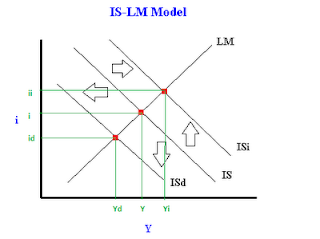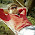Mathematically solving for equilibrium Y and I after a shift in the IS/LM model - FreeEconHelp.com, Learning Economics... Solved!

## 4/4/12

This post goes over some mathematical manipulations of the IS/LM model.  It also briefly discusses some of the economic intuition behind the equilibrium and possible shifts:

Suppose the economy can be summarized by the following set of equations:
C = 20 + 0.9Yd – 10(i - 0.1); I = 20 – 10(i - 0.1); G = 20; T = 10 + 0.2Y
Yd = Y - T
Md/P = 0.6Y – 200i
Ms =10,000

We are going to solve the IS and LM equations in terms of Y (and i) and then solve for the equilibrium price, consumption and investment level as well.  After this we will change an exogenous variable simulating expansionary fiscal policy and go through the implications.
First, let’s write out the equation for the closed economy IS curve:
Y = C + I + G
Or (by plugging in the Yd into the C, and the C, I, and G into the Y)
Y = 20 + 0.9(Y - 10 - 0.2Y) – 10(i-0.1) + 20 – 10(i – 0.1) +20

Multiply out terms (The Y's and the 50's) to get:
Y = 20 +0.9Y – 9 – 0.18Y -10i + 1 + 20 – 10i + 1 +20

Combining terms (or solving for Y) gives us:
Y – 0.9Y + .18Y = 53 -20i    or
0.28Y = 53 - 20i

Dividing both sides by 0.28 will give us the equation for the IS curve:
Y = 189.3 – 71.5i

Now solve for I to get the slope of the IS curve (economics has a weird way of plotting dependent vs independent variables!).
i = 2.65 - Y/71.5

Note that P doesn’t enter the IS equation, so this equation holds for any given P (price level).  P enters in the LM equation, and changes in P will shift the LM curve which will intersect the IS curve at different locations thus our IS curve holds for any given P.

LM equation is Ms/P=L(i,Y)or Md/P

Plugging in the associated values we get:
1,000/P = 0.6Y – 200i

And solving for Y (by adding 200i to both sides and dividing both sides by 0.6Y) we get:
0.6Y = (1,000/P) + 200i
Y = 1,666/P + 333i which is our LM equation, and it can be plotted by solving for i:
i = -5/P + Y/333
Note that P (price level) enters in the intercept term, which is why the LM curve shifts when P changes.

Now we are given equilibrium income (Y) of 180.  Plugging this into our IS equation will give us an i (interest rate) of:
i =  2.65 – 180/71.5 = 2.65 - 2.51  = 0.14

Now that we have equilibrium Y (given to us) and equilibrium i (which we solved for) we are able to calculate what equilibrium consumption and investment ought to be:

Plugging Y into our C (consumption) equation yields:
C = 20 + 0.9(Y - 10 - 0.2Y) – 10(i-0.1)  or
C = 20 + 0.9(180 - 10 - 0.2(180)) - 10(.14 - 1)
Multiplying out everything leaves us with:
C = 20 +  162 - 9 -32.4 - 1.4 + 1
C =140.2

And plugging Y into our investment (I) equation gets us:
I = 20 - 10(i - 0.1)
I = 20 - 10(0.14) + 1
I = 20 - 1.4 + 1
I =19.6
Finally, plugging i and Y into our LM equation gives us:
.14 = -5P + 180/333 We can now multiply both sides by P to get:
.14P = -5 + .54P and adding 5 and subtracting .14P from both sides gives:
5 = .4P now divide both sides by .4 to get:
P =12.5

Now we can simulate expansionary fiscal policy by increasing G by 11.2.  Note that a change in G (an exogenous variable) will shift the IS curve to the right.  The IS curve shifts right because an increase in G is a form of expansionary fiscal policy.  We now have to calculate all of the new equilibrium values.

There are two ways to do this, one is to do the whole problem over again from the beginning and calculating all new equilibrium values, or we can begin by looking at our original IS equation (the one we solved for earlier):

Y = 189.3 - 71.5i and add 40 (11.2/0.28 which is essentially the multiplier effect) to the intercept to account for the increase in G:
Y = 239.3 - 71.5i

This method works because we are just changing the equation for an exogenous variable that doesn't depend on either Y or i.

And we can set this equation equal to our LM equation from before (and we can do this because P has not changed and the LM curve has not shifted).

So IS = LM = 239.3 - 71.5i = 133.3/12.5 +333i  and we add 71.5i and subtract 133.3 from both sides to get:
106 = 404.5i and divide both sides by 404.5 to get:
i = .26Which will be our new equilibrium interest rate (note that it is higher, which is what we would expect because of the need to borrow more money and the crowding out effect).

Now we plug this equilibrium interest rate into our IS and LM equations to figure out equilibrium Y and we get:
220.71 which is higher than our previous Y which is what we would expect.

Finally, we can repeat the steps above for calculating the new levels of investment, and consumption.  We have just mathematically gone through a shift in the IS curve, let me know if you have questions or comments below.

1.Anonymous04 April

Thanks!!!

2.Anonymous04 April

I think the main part of solving this was making the connection between an increase in G and the IS curve while the LM curve remained the same and therefore the price level is the same.
Thank you

3.freeeconhelp05 April

You are absolutely right, there are two ways to help figure that out:

1) Fiscal policy (a change in G) only shifts the IS curve,
and
2) The LM equation has no mention of G

Thanks for the comment!

4.while solving is-lm eqnts,is it mandatory to solve for "R" frst and then I or we can dirctly solve "I" by solving d 2 eqnts